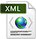Overall Objectives
Partnerships and Cooperations
Dissemination
BibliographyPDF e-Pub

## Section: New Results

### Hybrid Systems Modeling

Participants : Albert Benveniste, Benoît Caillaud.

#### Type-Based Analysis of Causality Loops In Hybrid Systems Modelers

Explicit hybrid systems modelers like Simulink / Stateflow allow for programming both discrete- and continuous-time behaviors with complex interactions between them. A key issue in their compilation is the static detection of algebraic or causality loops. Such loops can cause simulations to deadlock and prevent the generation of statically scheduled code. We have addressed this issue for a hybrid modeling language that combines synchronous Lustre-like data-flow equations with Ordinary Differential Equations (ODEs)  ,  . We introduce the operator $\text{last}\left(x\right)$ for the left-limit of a signal $x$. This operator is used to break causality loops and permits a uniform treatment of discrete and continuous state variables. The semantics relies on non-standard analysis, defining an execution as a sequence of infinitesimally small steps. A signal is deemed causally correct when it can be computed sequentially and only progresses by infinitesimal steps outside of discrete events. The causality analysis takes the form of a simple type system. In well-typed programs, signals are proved continuous during integration and can be translated into sequential code for integration with off-the-shelf ODE solvers. The effectiveness of this system is illustrated with several examples written in Zélus  (http://zelus.di.ens.fr ), a Lustre-like synchronous language extended with hierarchical automata and ODEs.

#### Semantics of multi-mode DAE systems

Hybrid systems modelers exhibit a number of difficulties related to the mix of continuous and discrete dynamics and sensitivity to the discretization scheme. Modular modeling, where subsystems models can be simply assembled with no rework, calls for using Differential Algebraic Equations (DAE). In turn, DAE are strictly more difficult than ODE. They require sophisticated pre-processing using various notions of index before they can be submitted to a solver. We have studied some fundamental issues raised by the modeling and simulation of hybrid systems involving DAEs  . The objective of this work is to serve for the evolution and the design of future releases of the Modelica language for such systems. We focus on the following questions:

• What is the proper notion of index for a hybrid DAE system?

• What are the primitive statements needed for a DAE hybrid systems modeler?

The differentiation index for DAE explicitly relies on everything being differentiable. Therefore, generalizations to hybrid systems must be done with caution. We propose to rely on non-standard analysis for this. Non-standard analysis formalizes differential equations as discrete step transition systems with infinitesimal time basis. We can thus bring hybrid DAE systems to their non-standard form, where the notion of difference index can be firmly used. From this study, general hints for future releases of Modelica can be drawn.